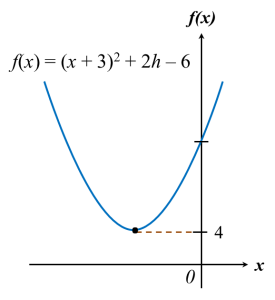# 3.8.3 Quadratic Functions, SPM Practice (Short Question)

Question 5:
Find the range of values of k if the quadratic equation 3(x2kx – 1) = kk2 has two real and distinc roots.

Solution:Question 6:
Given that the quadratic equation hx2 – (h + 2)x – (h – 4) = 0 has real and distinc roots.  Find the range of values of h.

Solution:Question 7:
The diagram below shows the graph of the quadratic function f(x) = (x + 3)2 + 2h – 6, where h is a constant.(a) State the equation of the axis of symmetry of the curve.
(b) Given the minimum value of the function is 4, find the value of h.

Solution:
(a)
When x + 3 = 0
x = –3
Therefore, equation of the axis of symmetry of the curve is x = –3.

(b)
When x + 3 = 0, f(x) = 2h – 6
Minimum value of f(x) is 2h – 6.
2h – 6 = 4
2h = 10
h = 5